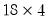Command Reference : Matrix Language Reference

 @subextract
Extract submatrix from matrix object.
Syntax: @subextract(m, n1, n2[, n3, n4])
m: matrix, vector, sym
n1: integer
n2: integer
n3: (optional) integer
n4: (optional) integer
Return: matrix
Returns a submatrix of a specified matrix, m.
The required arguments n1 and n2 are the row and column of the upper left corner of the region to be extracted.
The optional arguments n3 and n4 provide the row and column of the lower right corner of the region to be extracted.
If n3 or n4 are not provided, the corresponding element will be the last row or column of the source matrix.
Note that in some circumstances, you may find it easier to use the newer “.sub”, “.row”, and “.col” object data member functions. See “Matrix Data Members” “Sym Data Members” and the examples below.
Examples
matrix m1 = @mnrnd(20, 5)
matrix m1a = @subextract(m1, 3, 2)
extracts thematrix from the lower right corner of M1 starting at row 3 and column 2, while
matrix m1b = @subextract(m1, 1, 1, 5, 4)
extracts the upper left corner of M2 up through row 5 and column 4.
matrix m1c = @subextract(m1, 3, 2, 7, 3)
extracts the subtract from rows 3 to 7 and columns 2 to 3.
The commands
matrix m1d = @subextract(m1, 3, 1, 3)
vector v1d = @rowextract(m1, 3)
both extract row 3 from the matrix, while
matrix m1e = @subextract(m1, 1, 4, @rows(m1), 4)
vector v1e = @columnextract(m1, 4)
extract column 4. Note that
For illustration purposes, we repeat the previous commands followed by data member functions to perform equivalent extractions:
lower corner extraction,
matrix m1a = @subextract(m1, 3, 2)
matrix m1a_1 = m1.@sub(@range(3, m1.@rows), @range(2, m1.@cols))
upper corner extraction,
matrix m1b = @subextract(m1, 1, 1, 5, 4)
matrix m1b_1 = m1.@sub(@range(1, 5), @range(1, 4))
arbitrary rectangle extraction
matrix m1c = @subextract(m1, 3, 2, 7, 4)
matrix m1c_1 = m1.@sub(@range(3, 7), @range(2, 4))
row extraction as rowvector
matrix m1d = @subextract(m1, 3, 1, 3)
matrix m1d_1 = m1.@sub(3, @range(1, m1.@cols))
vector v1d = @rowextract(m1, 3)
vector v1d_1 = @transpose(m1.@row(3))
column extraction
matrix m1e = @subextract(m1, 1, 4, @rows(m1), 4)
matrix m1e_1 = m1.@sub(@range(1, @rows(m1)), 4)
vector v1e = @columnextract(m1, 4)
vector v1e_1 = m1.@col(4)
Cross-references